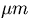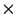Next: Z.Production:CDC_VTX;Track_Parameter Up: Banks in the Event Previous: Production:HDC;Cluster_to_Cell

## G1

Compressed Generator:Particle_List'' bank. The subroutine SQUZEV creates the data. DSSQUZ is the describe for this routine.

Element# 		: 		 1
Contents 		: 		 Number of valid words of correspondingGenerator:Particle_List'' bank.
It depends on the number of daughters, etc.
Format 		: 		  I*2, Number of I*2 words is equal to the number of
elements(particles) of Generator:Particle_List''.



IBUF(1)=Élement number of first cell.


Meanings of the values are as follows:

• [=8 : ] There is no daughter particles and the creation coordinates of the particle is less than 10from the interaction point(IP).
• [=9 : ] There are daughter particle(s) and the creation coordinates of the particle is less than 10from the interaction point(IP).
• [=11 : ] There are no daughter particles and the creation coordinates of the particle is greater than 10away from the interaction point(IP).
• [=12 : ] There are daughter particle(s) and the creation coordinates of the particle is greater than 10away from the interaction point(IP).
• [=14 : ] There are daughter particle(s) and particle has finite life time.

• [Element# :] 2
• [Contents :] Compressed Generator:Particle_List'' information.
• [Format :] I*2, I*4, R*4 mixed. All elements of Generator:Particle_List'' are packed in a single element data. The total sum of the values in the element number 1 is equal to the word length of this bank element. The original Generator:Particle_List'' information is compressed as follows.


NW=8 case:
HBUF(1,1)=RBUF(1):Particle serial number (RBUF(1))
(2,1)=RBUF(14):Pointer to the mother track. Negative when pointing
the bank Spring:Parton_List''
RBUF(2)=RBUF(2):Particle ID of PDG format.
(3-4)=RBUF(3-4):Particle mass (GeV) and charge.
(5-8)=RBUF(5-8):px, py, pz, and E (GeV).



NW=9 case:
HBUF(1,1)=RBUF(1):Particle serial number (RBUF(1))
(2,1)=RBUF(14):Pointer to the mother track. Negative when pointing
the bank Spring:Parton_List''
RBUF(2)=RBUF(2):Particle ID of PDG format.
(3)=RBUF(3):Particle mass.
HBUF(1,4)=RBUF(4):Particle charge.
(2,4)=RBUF(12):number of daughter particles.
RBUF(5-8)=RBUF(5-8):px, py, pz, and E (GeV).
HBUF(1,9)=RBUF(13):Pointer to the daughter particle.
(2,9)=RBUF(14):Pointer to the mother, same as the one stored in HBUF(2,1).



NW=11 case:
HBUF(1,1)=RBUF(1):Particle serial number (RBUF(1))
(2,1)=RBUF(14):Pointer to the mother track. Negative when pointing
the bank Spring:Parton_List''
RBUF(2)=RBUF(2):Particle ID of PDG format.
(3)=RBUF(3):Particle mass.
(4)=RBUF(4):Particle charge.
(5-8)=RBUF(5-8):px, py, pz, and E (GeV).
(9-11)=RBUF(9-10):x, y, and z (cm) coordinates of
particle creation point.



NW=12 case:
HBUF(1,1)=RBUF(1):Particle serial number (RBUF(1))
(2,1)=RBUF(14):Pointer to the mother track. Negative when pointing
the bank Spring:Parton_List''
RBUF(2)=RBUF(2):Particle ID of PDG format.
(3)=RBUF(3):Particle mass.
HBUF(1,4)=RBUF(4):Particle charge.
(2,4)=RBUF(12):number of daughter particles.
(5-8)=RBUF(5-8):px, py, pz, and E (GeV).
(9-11)=RBUF(9-10):x, y, and z (cm) coordinates of
particle creation point.
HBUF(1,12)=RBUF(13):Pointer to the daughter particle.
(2,12)=RBUF(14):Pointer to the mother, same as the one



NW=14 case:
HBUF(1,1)=RBUF(1):Particle serial number (RBUF(1))
(2,1)=RBUF(14):Pointer to the mother track. Negative when pointing
the bank Spring:Parton_List''
RBUF(2)=RBUF(2):Particle ID of PDG format.
(3)=RBUF(3):Particle mass.
HBUF(1,4)=RBUF(4):Particle charge.
(2,4)=RBUF(12):number of daughter particles.
(5-8)=RBUF(5-8):px, py, pz, and E (GeV).
(9-11)=RBUF(9-10):x, y, and z (cm) coordinates of
particle creation point.
HBUF(1,12)=RBUF(13):Pointer to the daughter particle.
(2,12)=RBUF(14):Pointer to the mother, same as the one.
RBUF(13)=RBUF(16):Particle life time (cnsec).
(14)=RBUF(17):Decay length(cm).Next: Z.Production:CDC_VTX;Track_Parameter Up: Banks in the Event Previous: Production:HDC;Cluster_to_Cell
Miyamoto Akiya
11/20/1998